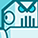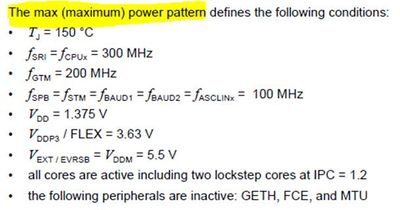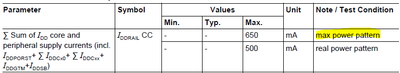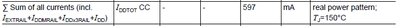Announcements

# AURIX™ Forum DiscussionsLevel 1
Level 1# Max current consumption of SAK-TC364DP-64F300F

Hello,

As per below max power pattern,Can you pls confirm the current consumption (IDD) at VDD level = 1.375V will be as below for max pattern?Also, its mentioned that sum of all currents = 597mA (for real power pattern) as below –Does it includes all current at Vext = 5.5V, Vdd = 1.375V & Vddp3 = 3.63V ?

Can you pls let us know what is the “Sum of all currents @ for max power pattern” ?

In brief (as per datasheet) –

At Vext = 5.5V, max power pattern current consumption will be 44 mA,

At Vdd = 1.375V, max power pattern current consumption will be 650 mA,

At Vddp3 = 3.63V, max power pattern current consumption will be 45 mA.

Regards,

Abhishek D.

0 Replies